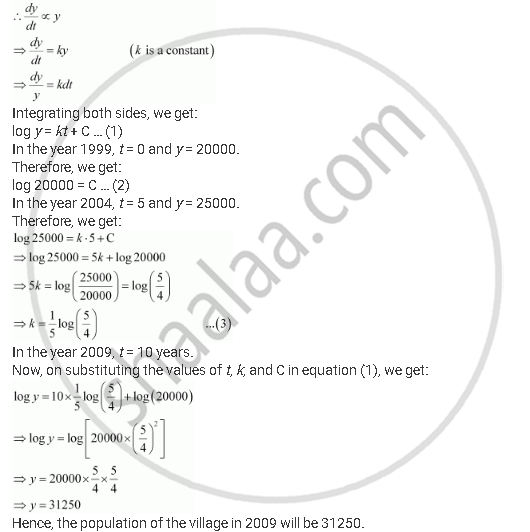Share

# The Population of a Village Increases Continuously at the Rate Proportional to the Number of Its Inhabitants Present at Any Time. If the Population of the Village Was 20000 in 1999 and 25000 in the Year 2004, What Will Be the Population of the Village in 2009? - CBSE (Science) Class 12 - Mathematics

ConceptMethods of Solving First Order, First Degree Differential Equations Linear Differential Equations

#### Question

The population of a village increases continuously at the rate proportional to the number of its inhabitants present at any time. If the population of the village was 20000 in 1999 and 25000 in the year 2004, what will be the population of the village in 2009?

#### Solution

Let the population at any instant (t) be y.

It is given that the rate of increase of population is proportional to the number of inhabitants at any instant.Is there an error in this question or solution?

#### APPEARS IN

NCERT Solution for Mathematics Textbook for Class 12 (2018 to Current)
Chapter 9: Differential Equations
Q: 15 | Page no. 421

#### Video TutorialsVIEW ALL 

Solution The Population of a Village Increases Continuously at the Rate Proportional to the Number of Its Inhabitants Present at Any Time. If the Population of the Village Was 20000 in 1999 and 25000 in the Year 2004, What Will Be the Population of the Village in 2009? Concept: Methods of Solving First Order, First Degree Differential Equations - Linear Differential Equations.
S